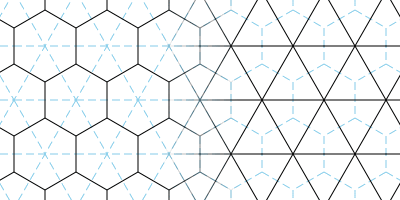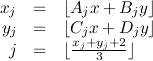## Monday, April 23, 2007

### Hex grids

Geometry

A tiling of regular hexagons can be laid out on a rectangular grid:Via the Pythagorean Theorem, the relationship between horizontal and vertical grid line spacing is:For every hexagonal tiling there is an isomorphic triangular tiling representing hexagon adjacencies:Coordinates

How should coordinates be assigned to the hexagons in the grid? The first thing many people think of is to try to approximate the rows and columns of a rectangular grid:Transforming the isomorphic triangle grid to rectangular form shows the problem with this, though:Because the diagonals between adjacent hexes run in different directions on alternate rows, working with the hex grid is more complicated. For instance, a pathfinding routine needs to know the coordinates of each hex's neighbors. With this layout it is necessary to see if the hex is on an even row or an odd row, and generate a different set of neighbor coordinates for each.

A better way to number hexes is to keep both horizontal and “vertical” axes straight:Now, the transformation between the hexagonal layout and the square layout preserves all straight lines, and each hex looks like every other:It's fairly easy to convert back and forth between the two coordinate systems if the first one is desired from an interface standpoint.

Converting from plane coordinates to hex coordinates

How can a position in the continuous Cartesian plane be converted into hex grid coordinates? Examine this affine transformation:Four of each hex's edges now line up with the unit grid, and the two remaining diagonal edges are between hexes with the same X coordinate. Thus, every point within a square on the unit grid corresponds to the same X coordinate in the hex grid. After transforming the input point, round each component down (the floor() function in C) to get the integer coordinates of a unit grid square. From these unit grid square coordinates compute the hex X coordinate by summing the grid square X and Y, adding 2 (or whatever offset is necessary given your hex layout), and integer-dividing by 3, since the band of squares that correspond to the same hex X coordinate is 3 grid squares wide. In equation form:The hex Y coordinate is determined via a similar method. Using a different affine transform, once again align the hexes such that four of the hex edges are aligned with the unit grid, but this time ensure diagonal edges are between hexes with the same Y coordinate:Again, determine integer grid square coordinates by rounding down the transformed point's coordinates, then sum them, add 2, and integer-divide by 3 to yield the hex's Y coordinate.The precise A, B, C, and D coefficients for each of the transforms will depend on the scale and geometry of your hex grid.

To build a transformation matrix, think about what you want to come out when you put in (1, 0) and (0, 1). (1, 0) becomes (A, C) with our equations, while (0, 1) becomes (B, D).

Distance

Given the signed offset of a hex in x and y (in integer grid coordinates), it's easy to compute the number of steps needed to move to it. The formula is slightly different depending on which direction the grid's diagonals go.

If (1, 0) and (0, 1) are adjacent in the hex grid:If (0, 0) and (1, 1) are adjacent in the hex grid:References

Amit Patel has an excellent site covering lots of game-related topics, including a section on hex grids.Anonymous said...

Very nice tutorial!Anonymous said...

Nice, but could be a little clearer

where does
A(i/j)x B(i/j)y C(i/j)x D(i/j)y

come from?

Unknown said...

Can you clarify this post a little bit? Its a great solution, but I can't quite figure out the two affine transforms. The lower one is a shear, but what's the upper one?

This is the cleanest solution to hex hit-testing by far, and its killing me that I can't figure out the matrix!

Thanks.

James McNeill said...

How do you come up with the matrix

[ A B ]
[ C D ] ?

Let's define some desired outputs and figure out what their inputs should be. With a couple of these we can solve for the matrix coefficients.

I'll assume that the input hex grid scale has scale [r, s] where r is the distance from the center of a hex to an edge, and s is the distance from the center of a hex to a corner. In the top diagram this would be [sqrt(3), 2]. If your hexes have extent measured in pixels or whatever you'll supply the appropriate values for [r, s]. Note that you can scale hexes non-uniformly, too, for instance if you are presenting an isometric view. Put the origin at the center of a hex.

Examine the affine transformation above with the red lines. On the output grid, a vector of [1, 0] corresponds to the input vector [0, -s]. Likewise the output vector of [0, 1] corresponds to the input vector [r, s/2].

Plug the first relationship into our matrix multiplication equation:

A * 0 + B * -s = 1
C * 0 + D * -s = 0

This lets us solve for a couple of the matrix coefficients:

B = -1/s
D = 0

Now plug these in along with the second relationship:

A * r - 1/s * s/2 = 0
C * r + 0 * s/2 = 1

This lets us solve for the last two coefficients:

A = 1/2r
C = 1/r

The complete matrix:

x' = 1/(2*r) * x - 1/s * y
y' = 1/r * x

This matrix is used to solve for the X hex grid coordinate. You go through a similar process with the green diagram above to produce a matrix for determining the Y hex grid coordinate. In this case the green diagram indicates these mappings:

[r, s/2] --> [1, 0]
[-r, s/2] --> [0, 1]

Unknown said...

Holy mackerel, it works. Thank you for reminding me of the proper way to derive a transformation matrix!

I've implemented this algorithm in python/numpy. http://gist.github.com/583180 I hope someone finds it useful.

FWIW, I derived the two transformation matrices XM and YM using matrix inversion (mathematically equivalent way of solving the same linear system).

This is really a great algorithm.

James McNeill said...

Cool! Glad it worked; glad to see it's finding use. I enjoyed reading your Python implementation. I use Python at work a fair amount but I have not gotten into numpy yet.

I have a hunch that it might be possible to shave out one of the floor operations by combining the math for X and Y.

Unknown said...

I would like to second what Eric said, that this is a really great algorithm! Also a bazillion thanks must go to Eric for sharing his code, I am trying to implement this kind of thing in java and constructing the transforms is a bit above me. Luckily your python implementation is quite understandable to a java person ;)

There is one burning question though: when you say "adding 2 (or whatever offset is necessary given your hex layout)", I don't understand how the hex layout would affect what you should add, would you be happy to clarify it a bit please? (In Eric's code I am assuming the value of R = 2 is what you're talking about there?) - My thought for this is that if the top left hex has coordinates 0,0 this offset should be 0 for x and 0 for y?

Thanks!

James McNeill said...

I don't think I had thought out the "add 2 or whatever is appropriate" comment too much when I wrote it. But let's say you were to do the red diagram transform so the diagonals stayed pointing from upper-right to lower-left, which would be less of a rotation than the one I did. In that case, your lines of constant hex X would run southwest to northeast, so you'd need something of the form floor((x - y + n) / 3) instead of floor((x + y + n) / 3). If you look at how the hexes lie on that grid, you need coordinates (0, 0), (1, 0), and (2, 0) to all map to x=0, so in this case n=0. In the red diagram as it stands in the article, you need (-2, 0), (-1, 0), and (0, 0) to map to x=0, so n=2.

I left the original article vague on specifics because people lay out their hex grids in different ways. For instance you can rotate the grid by 90 degrees so that you get vertical columns (instead of horizontal rows). Or you might have a coordinate system with Y increasing as you go downward, instead of Y increasing as you go upward. The specific numbers are different for these cases but the principles remain the same.Anonymous said...

I think there may be an issue with the python code or I'm missing something in my code. If I'm converting negative hexagonal values to cartesian space they are only correct if I remove the offset with a conditional statement.

Here's my Java hack… would love a mathematical solution just to be more succinct.

https://gist.github.com/1150829

James McNeill said...

"Works differently with negative numbers" problems can usually be traced back to the fact that languages in the C/Java family convert floating-point numbers to integers by truncating toward zero instead of rounding.

In this case, your floor operations in the lines defining j and k are superfluous (I think) because the math is all integer math. And integer math will truncate: 1/3 == 0, but -1/3 == 0 too, instead of -1 which is what we're after.

If you were to convert floorDot() to return a PVector instead of an HVector (and thus to do everything in floating-point instead of integer) I think you could probably make things work without the hacks. Something like:

PVector floorDot(PVector v, float[][] mtx) {
float j = floor(mtx*v.x + mtx*v.y);
float k = floor(mtx*v.x + mtx*v.y);
return new PVector(j,k);
}

Hope this helps!Anonymous said...

Lovely. Works perfectly now.
Tried (int) instead of floor() and had the same issue so I've kept the floor in there.
Sincere thanks at 3am.

James McNeill said...

Yeah, sorry I wasn't clear. You need to keep the floor() calls; I was just saying that if you had all-integer math inside them then they weren't doing anything. Now that the math inside them is floating-point they are working as intended.

pablo said...

It's all very nice. James, could I use one or two of your figures in a scientific article? I would give appropriate reference to the source, of course.

James McNeill said...

Pablo,

Sure, feel free to use the diagrams.

Unknown said...
This comment has been removed by a blog administrator.
Evan said...

Your answer is ok. What is the software you used to create this matrix? was it creately ?

James McNeill said...

If I remember correctly, I used Python scripts to generate SVG for the diagrams, which I then rendered using Inkscape and finished in the GIMP.

Unknown said...

Hey,

Thanks for keeping the tutorial up its helping! :D

SC said...

I'm still too dumb to understand how to use this information to make a world point to hex grid and vice versa in code.

Unknown said...

I have a grid where the hexes are oriented so that the flat side is horizontal instead of vertical. The tile at [0, 0] is also located at the "top center". Positive x extends down to the right and positive y down to the left. Like in this image: https://photos.app.goo.gl/2JfweiCVAmiC5iEv8

I can't seem to figure out the transformation matrices for the affline transforms. Any help is much appreciated.
Thanks!